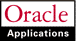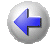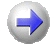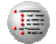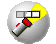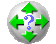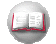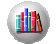Previous Next Contents Index Navigation Glossary Library

## Calculating Budget Amounts

You can implement Oracle Projects to calculate the raw and burdened costs and the revenue amounts for each budget line based on the quantity or raw cost that you enter, and based upon the rules that you define. You define rules using budget calculation extensions. A budget calculation extension is a client extension you write using PL/SQL.

Using the rules that you define in the budget calculation extension, the system calculates the amounts for each budget line at the following times:

• When you leave the quantity field, the raw cost and the burdened cost are calculated (for a cost budget).
• When you leave the raw cost field, the burdened cost is calculated, if the burdened cost is blank in the Budget Lines window.
• When you leave the quantity field in the Budget Lines window, the revenue amount is calculated (for a revenue budget).
• When you enter the Resource, Dates, and Quantity, or change the resource or dates for existing amounts, the system calls the extensions and recalculates any items specified for recalculation due to the changes.
Your calculation rules also specify whether a user can alter a calculated value, or must accept the calculated value.